# Multiplication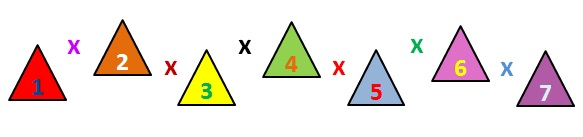It helps to know your times tables! Click on the times tables picture.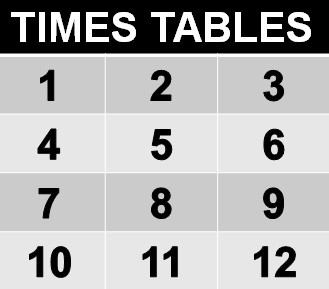## Muliplying by 10

A decimal point comes after a whole number e.g. 3.4 - the number three is a whole number.

Move the decimal point one place to the right.

## Example 1

2 x 10 = ?

Move the 2 from the units to the tens column.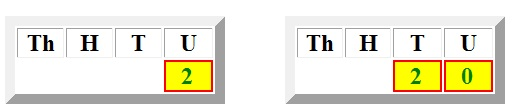## Example 2

8.3 x 10= ?

Move the 8 from the units to the tens column and .3 to the units column.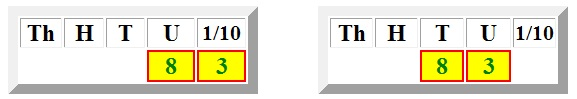## Muliplying by 100

Move the number or numbers two places to the right.

## Example 1

5 x 100 =?

Move the 5 from units to the hundreds column.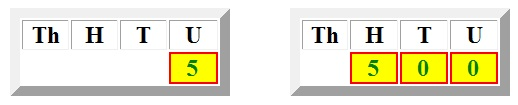## Muliplying by 1000

Move the decimal point three places to the right.

2.2 x 1000 = ?

Move the 2 from units to the thousands column and .2 to the hundreds column.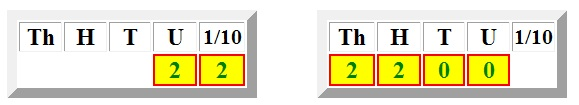# Muliplying Numbers

## Example 1

22 x 6 = ?

2 by 6 = 12
2. Enter 2 and carry 1 to the tens
3. Multiply the 2 in the tens coloumn
by 6 = 12
4. Plus the 1 carried over = 13
5. Enter 3 in the tens column and
carry 1 over to the hundreds column

ThHTU
22
6
132
1

x

## Example 2

43 x 11 = ?

1. Multiply 43 by 1 = 43
2. Multiply 43 by 10= 430
3. Add 43 + 430 = 473

ThHTU
43
11
43
430
473

x

## Example 3

343 x 32 = ?

1. Multiply 343 by 2 = 686
2. Multiply 343 by 30= 10,290
3. Add 686 + 10290= 10,976

ThHTU
343
32
686
10, 290
10, 976

x

# VIDEO

Have a look at the demonstration on the video to enhance your learning.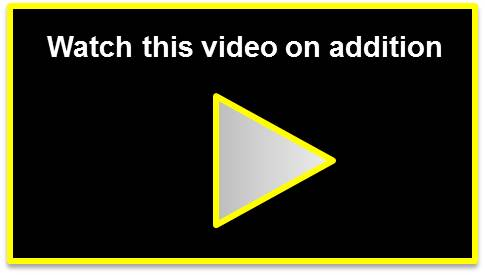Now try the Exercises!